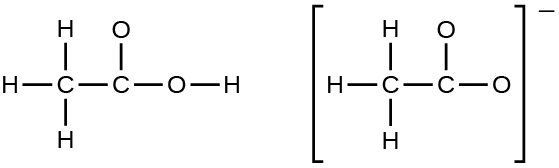# Problem: In terms of the bonds present, explain why acetic acid, CH 3CO2H, contains two distinct types of carbon-oxygen bonds, whereas the acetate ion, formed by loss of a hydrogen ion from acetic acid, only contains one type of carbon-oxygen bond. The skeleton structures of these species are shown:

###### FREE Expert Solution

We are being asked to differentiate the C-O bond of acetic acid (CH3CO2H) and acetate ion (CH3CO2-). We will have to draw the Lewis Structure of acetic acid (CH3CO2H) and acetate ion (CH3CO2-) first and determine if there are resonance structures possible.

To solve this problem we shall follow these general steps for each molecule:

Step 1: Determine the central atom of the molecule.

Step 2: Calculate the total number of valence electrons present.

Step 3: Draw the Lewis Structure.

Step 4: Identify resonance form or structures, if any.###### Problem Details

In terms of the bonds present, explain why acetic acid, CH 3CO2H, contains two distinct types of carbon-oxygen bonds, whereas the acetate ion, formed by loss of a hydrogen ion from acetic acid, only contains one type of carbon-oxygen bond. The skeleton structures of these species are shown: